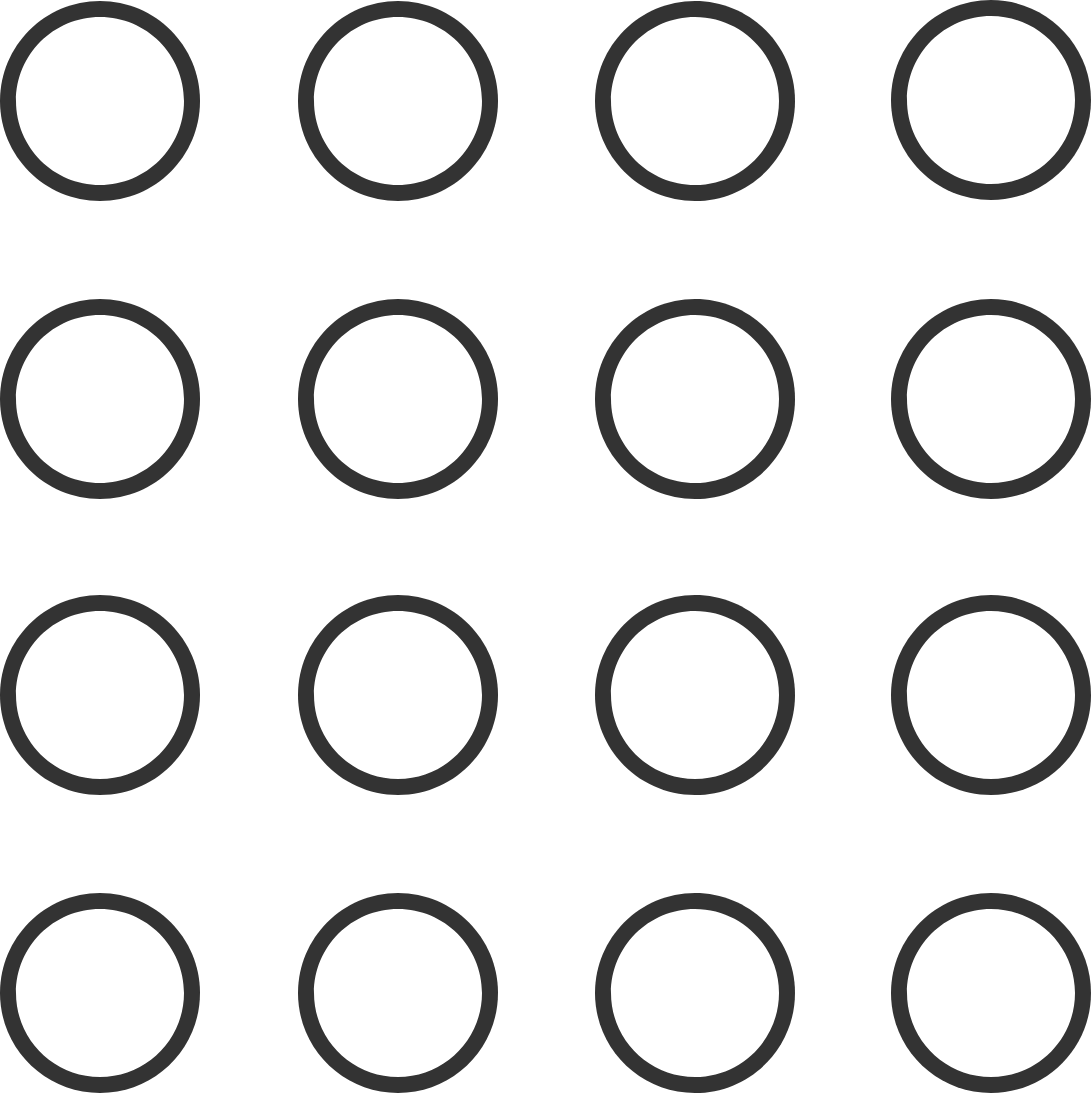# Christmas Streak 31/88: A Bit Farther

Probability Level 4Tom is jumping around a $4\times4$ grid of circles with the following conditions:

• He starts from any of the 16 circles.
• He always jumps in a straight line from one circle to any other circle.
• Each subsequent jump is farther than the last.
• He doesn't visit a circle already visited.

The maximum number of circles that he can step on is $m,$ and the number of ways of doing so is $r.$

Find the value of $mr.$


Note: The grid is fixed on the ground, so a method obtained by rotating another is considered different.

×